maths > construction-basics

what you'll learn...

overview

•  Properties of quadrilaterals is explained

•  The number of independent parameters in a quadrilateral is $1$$1$

•  For a given parameter, construction of quadrilaterals is approached as combination of triangles (sss, sas, asa, rhs, sal) and using the properties of quadrilaterals.

recap

•  sum of all interior angles is ${360}^{\circ }$${360}^{\circ}$. A quadrilateral is made of two triangles sharing one side. Each triangle is defined by $3$$3$ parameters and since they share a side, one parameter is common in the two sets of $3$$3$ parameters. Thus, a quadrilateral is defined by $5$$5$ parameters.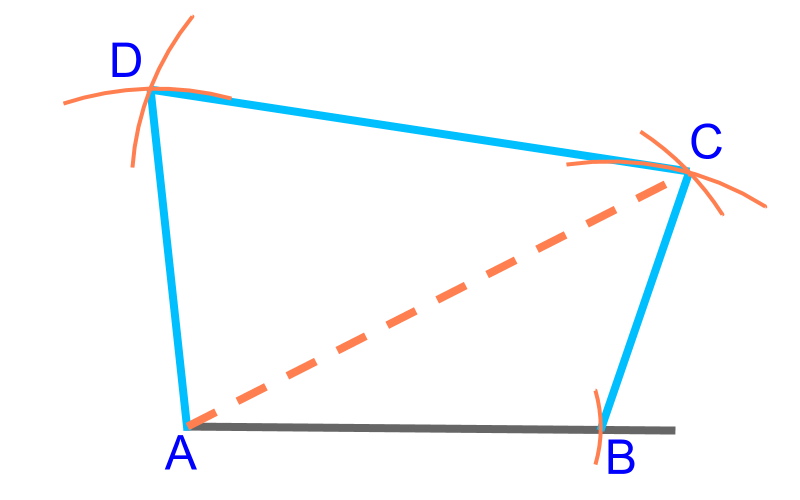To construct a quadrilateral, 4 sides ($\overline{AB}$$\overline{A B}$, $\overline{BC}$$\overline{B C}$, $\overline{CD}$$\overline{C D}$, $\overline{AD}$$\overline{A D}$ ) and a diagonal ($\overline{AC}$$\overline{A C}$) are given. This is illustrated in the figure. The quadrilateral can be constructed by considering this as two SSS triangles $ABC$$A B C$ and $ACD$$A C D$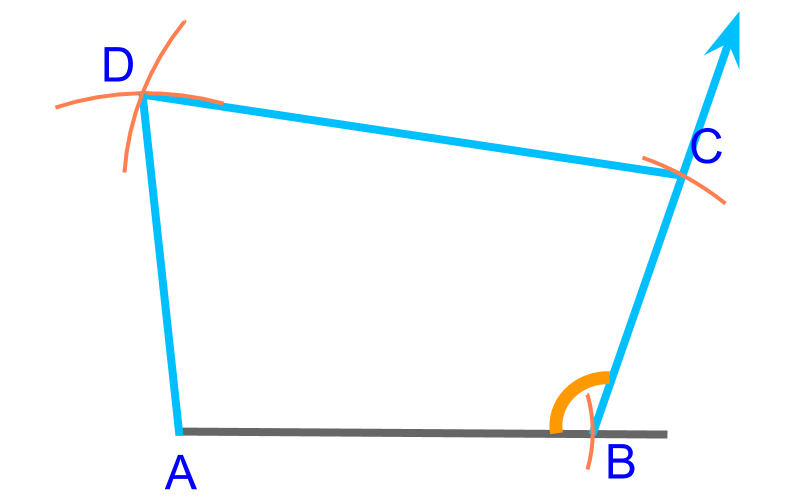To construct a quadrilateral, 4 ($\overline{AB}$$\overline{A B}$, $\overline{BC}$$\overline{B C}$, $\overline{CD}$$\overline{C D}$, $\overline{AD}$$\overline{A D}$ ) sides and an angle ($\angle B$$\angle B$) are given. This is illustrated in the figure. The quadrilateral can be constructed by considering this as an SAS triangle $ABC$$A B C$ and another SSS triangle $ACD$$A C D$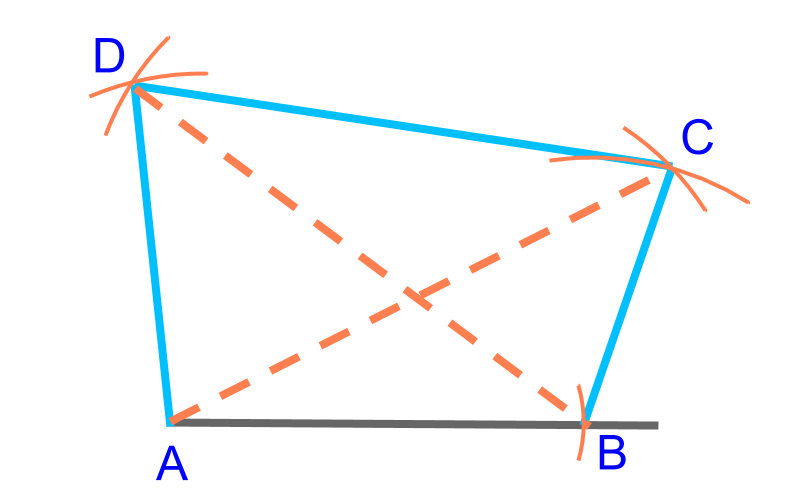To construct a quadrilateral, $3$$3$ ($\overline{AB}$$\overline{A B}$, $\overline{BC}$$\overline{B C}$, $\overline{AD}$$\overline{A D}$ ) sides and two diagonals ($\overline{AC}$$\overline{A C}$, $\overline{BD}$$\overline{B D}$) are given. This is illustrated in the figure. The quadrilateral can be constructed by considering consider this as two SSS triangles $ABC$$A B C$ and $ABD$$A B D$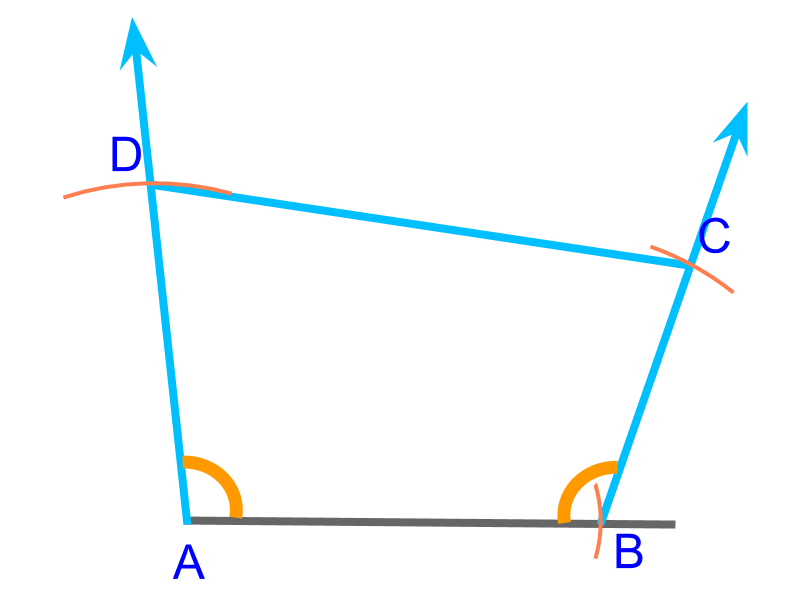To construct a quadrilateral, $3$$3$ sides ($\overline{AB}$$\overline{A B}$, $\overline{BC}$$\overline{B C}$, $\overline{AD}$$\overline{A D}$ ) and $2$$2$ angles ($\angle A$$\angle A$, $\angle B$$\angle B$) are given. This is illustrated in the figure. The quadrilateral can be constructed by considering this as two SAS triangles $ABC$$A B C$ and $BAD$$B A D$.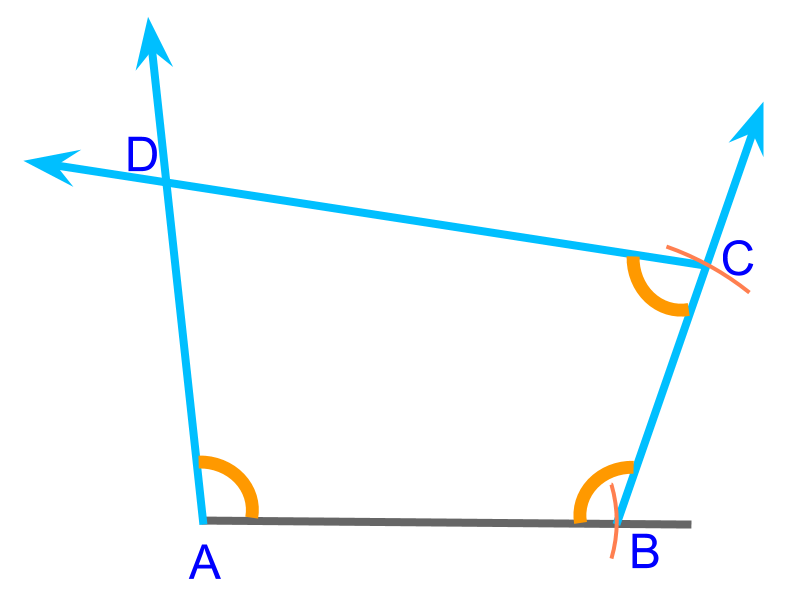To construct a quadrilateral, $2$$2$ sides ($\overline{AB}$$\overline{A B}$, $\overline{BC}$$\overline{B C}$ ) and $3$$3$ angles ($\angle A$$\angle A$, $\angle B$$\angle B$, $\angle C$$\angle C$)are given. This is illustrated in the figure. To construct, "consider this as an SAS triangle $ABC$$A B C$ and another ASA triangle with two angles $\angle C$$\angle C$ and $\angle A$$\angle A$". Note that $△ACD$$\triangle A C D$ is formed by angles $\angle C$$\angle C$ and $\angle A$$\angle A$

summary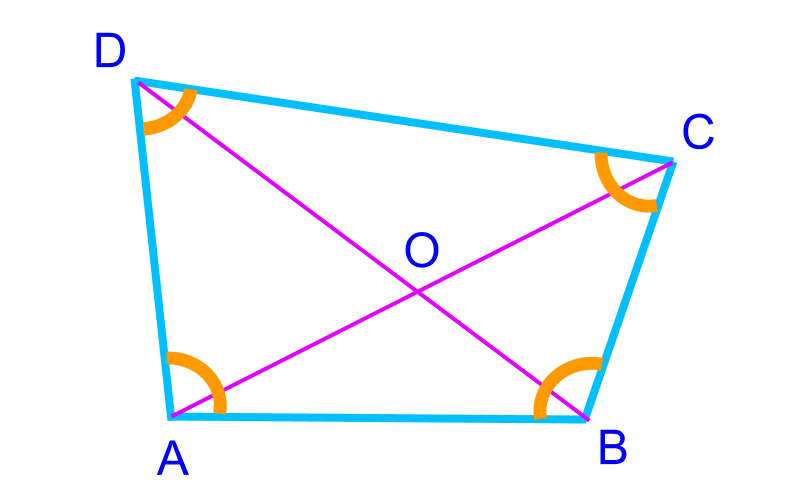•  sum of interior angles ${360}^{\circ }$${360}^{\circ}$

The formulations of questions

•  4 sides and a diagonal

•  3 sided and 2 diagonals

•  4 sides and an angle

•  3 sides and 2 angles

•  2 sides and 3 angles

Outline